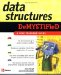Java s version of the stack-linked list is less complex than the C++ version because Java programmers use the Stack class defined in the java.util package. The Stack class contains the push() , pop() , and empty() member methods similar to those that you define in the C++ version of the stack-linked list. Understanding the basics of the C++ version helps you also understand the internal implementation of the stack, which in turn helps you make better choices when you select from data structures that have already been written, such as the Stack class in Java.

The following Java application is comparable to the C++ application that is discussed throughout this chapter. The application begins by declaring an instance of the Stack class and then calls the push() member method to place three values on the stack. The push() member method expects an object rather than an integer. Therefore, integers are passed to the constructor of the Integer wrapper class, as discussed in detail in the Linked Lists Using Java section of Chapter 6.

After the stack is loaded with data, the Java application determines if there is any data stored on the stack by calling the empty() member method of the Stack class. The empty() member method performs the same functionality as the isEmpty() member function in the C++ application.

The empty() member method returns a Boolean true if the stack is empty; otherwise , a Boolean false is returned. The program uses the not operator (!) to reverse the logic. That is, if the empty() member method returns false , then statements within the loop execute.

The first statement within the while loop calls the pop() member method, which returns an Integer wrapper class object. The pop() method returns an object, so this must be typecast to an integer. The value is displayed using the System.out.println() method. Here s what is displayed when you run this application:

30

20

10

` import java.util.*; public class StackLinkedListDemo {  public static void main(String[] args)  {  Stack stack = new Stack();  stack.push(new Integer(10));  stack.push(new Integer(20));  stack.push(new Integer(30));  while(!stack.empty())  {  Integer temp = (Integer)stack.pop();  System.out.println(temp);  }  } } `Data Structures Demystified (Demystified)
ISBN: 0072253592
EAN: 2147483647
Year: 2006
Pages: 90

Similar book on Amazon